# College Physics : Doppler Effect

## Example Questions

### Example Question #1 : Doppler Effect

Suppose that car A and car B are both traveling in the same direction. Car A is going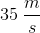and is sounding a horn with a frequency of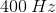. If car B is traveling at a speed of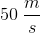in front of car A, what frequency of sound does car B hear?

Note: The speed of sound in air is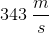.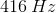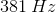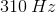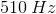Explanation:

The important concept being tested in this question is the Doppler effect. When a source of sound waves (or any other wave) is emitted from a source and is moving relative to some observer, the actual frequency of the wave will be different for the observer.

For starters, we'll need to use the Doppler equation.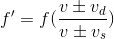The trickiest part about this question is to decide whether to add or subtract in both the numerator and denominator. To find the right sign orientation, it's helpful to do a quick thought experiment. Imagine that only one of them is moving and the other is stationary. Decide how that will affect the observed frequency; will it increase it or decrease it? Then repeat for the other one.

First, let's consider the detector (aka observer). We're told that car B, the detector, is traveling ahead of car A and also in the same direction. This means that car B is driving away from car A. So in this situation, is the frequency that car B hears expected to increase or decrease? The answer is that it will decrease. Since car B is traveling away from car A, each successive wave will take longer to reach car B. Hence, we will use subtraction in the numerator because that will make the observed frequency smaller.

Now let's apply this same logic to the denominator, which deals with the source of the sound waves. We know that car A is traveling in the same direction as car B and is behind. This means that car A is traveling toward car B. So from this perspective, each successive wave is expected to get closer together, thus making the time between each wave smaller and the frequency bigger. In the denominator, will adding or subtracting make the observed frequency bigger? The answer is subtraction. By making a smaller number in the denominator, the entire fraction becomes larger.

Keeping this information in mind, we'll need to use subtraction in both the numerator and denominator. Once we plug in the values given in the question stem, we have everything we need to solve for the answer.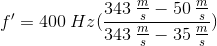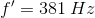### Example Question #1 : Doppler Effect

On planet Borg, a male insect is flying toward his mate at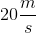while buzzing at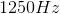. The female insect is stationary and is buzzing at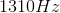. What is the speed of the sound on planet Borg?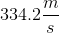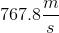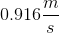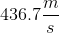Explanation: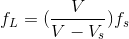Rearrange the equation by solving for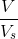to get: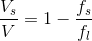Finally we plug in our known values and solve for: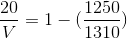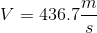### All College Physics Resources